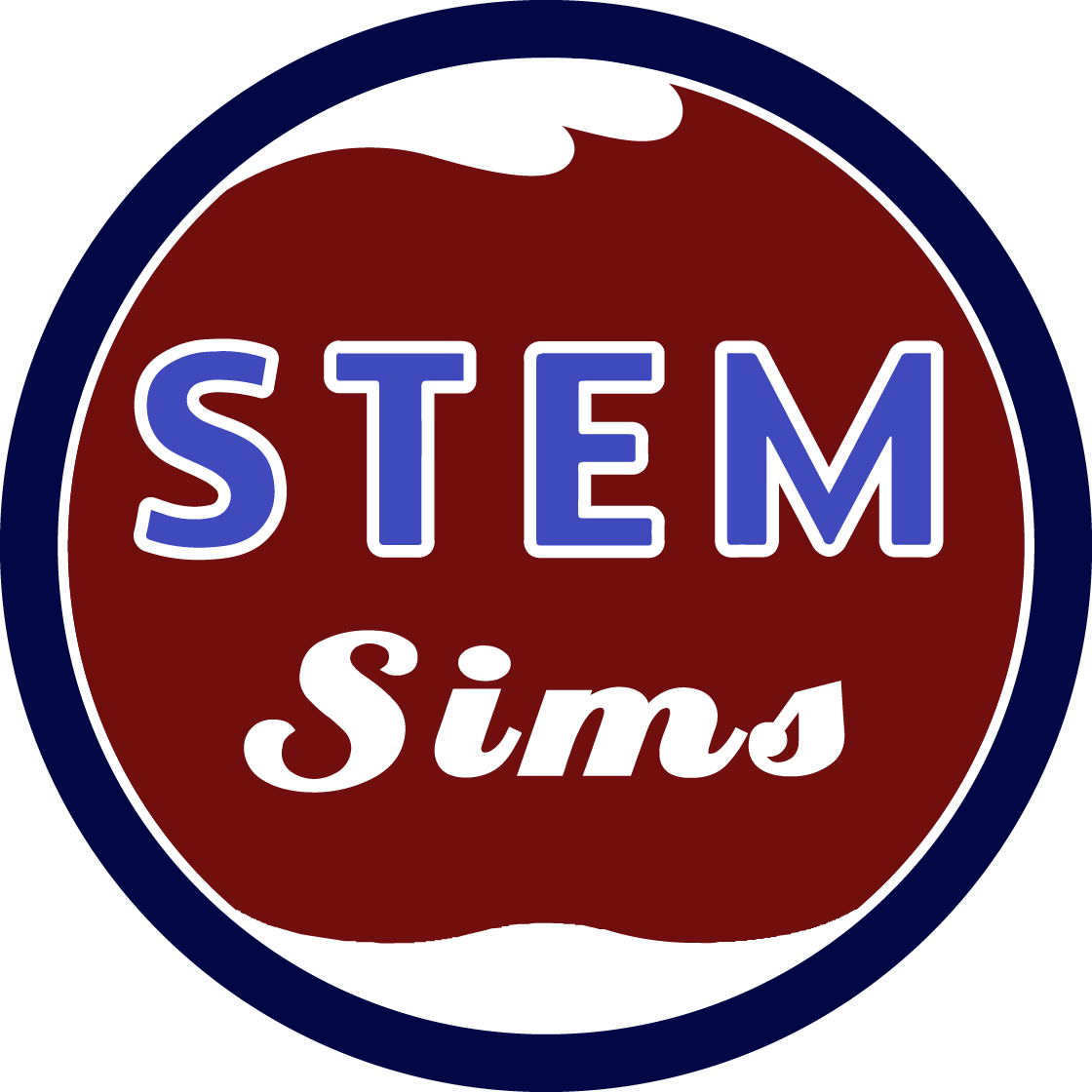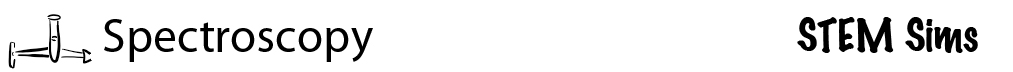PDF Lesson### Lessons 2: Fine-tuning Your Analysis of Solutions

In the previous lesson you estimated the unknown concentration of a solution by comparing values. In this lesson you’ll use some high-powered mathematics and graphical techniques to improve your ability to predict the unknown concentration of a solution.

### Doing the science

1. You must have complete Lesson 1 and have your complete set of data to begin this lesson.
2. Transfer your data from the table in Lesson 1 to Table 1 below. Please note that the order of the solutions’ concentrations in the data table has been reversed; so make sure that you record the correct voltage with the correct concentration.
3. In Lesson 1, the laser shot light through the solutions. Some light passed through and some was absorbed by the solution. The % Transmission is a measure of how much light passed through the sample. Calculate the % Transmission of each solution using the following equation and record your results in Table 1.

% Transmission for a specific solution =
voltage of a specific solutionvoltage of the 0% solution
x 100%

4. Absorbance is a measure of how much light is absorbed by a solution. Use the following equation to calculate the absorbance of each solution and record these values in Table 1. You’ll need a scientific calculator to complete this calculation.

Absorbance = log (voltage of the 0% solution/voltage of a specific solution)

5. Create a graph plotting the concentrations of the solutions on the "x" axis and absorbance on the "y" axis.
6. Use your graph to determine the concentration of Unknown A or B and record your results at the bottom of Table 1.

Table 1. Unknown Solution: